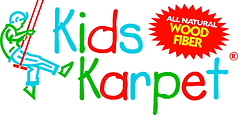Fill in one of the forms below to have the area and volume calculated for you.
See below for how to determine the shapes of your play area.

Area Calculations:

Draw your site as accurately as possible - graph paper works well.

For odd or complex shapes, divide the area into combinations of rectangles, triangles, and/or circles.
Use the following formulas to determine area:

Rectangle: Area = length x width
Circle: Area = 3.1416 x the square of the radius (distance from the center to any point on the circle)
Triangle: Area = 1/2 x length of base x length of height

You will need to install 16" of Kids Karpet Playground surface to achieve a depth of 12"

after allowing for a 4" compaction* post installation.

* The material will compact approximately 33% after it is first installed, requiring an additional 4" depth installed for each cubic yard of material.